# How to transpose cells in Microsoft Excel

In this article, we are going to learn all about Transpose function. What is the use of Transpose function and how we can transpose the cells?

In Microsoft Excel we have 2 ways to transpose the data?

We can transpose the cell from Vertical range to Horizontal range and Horizontal range to Vertical range.

• Transpose Function
• Transpose option

Let’s take an example and understand how we can use transpose function?

We have name list along with Credit number in 2 columns and we want to update it in row. How do we do it using Transpose function?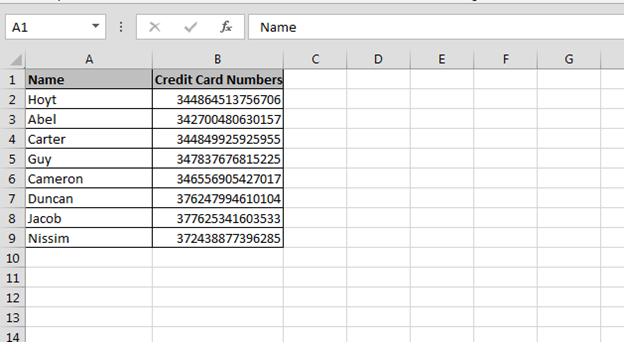• First count cells in the ranges -it’s 8.
• Then select 8 cells in 2 vertical range; refer below image:-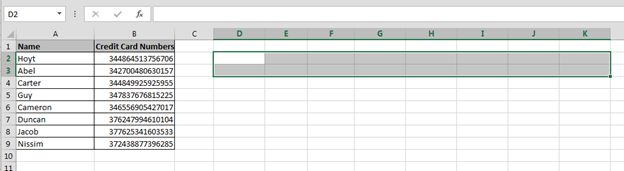• Press the key F2.
• Enter the function =TRANSPOSE(A2:B9)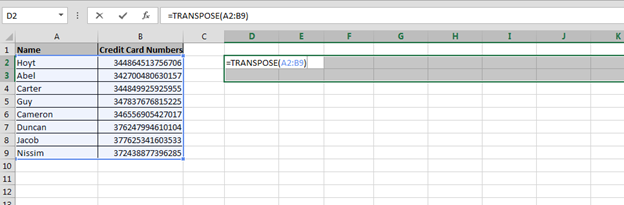• Press Ctrl+Shift+Enter.
• Columns text will get updated in the selected rows.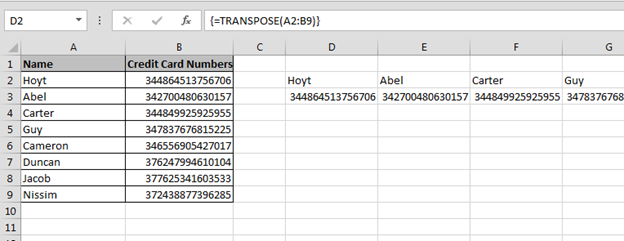In this way, we use Transpose function in Microsoft Excel.

How to use Transpose Option to shift the data from rows to columns and columns to rows?

You will find this option under the Paste Special option. Now we will see how we can utilize this option to shift the range position.

• Select the range that we want to convert.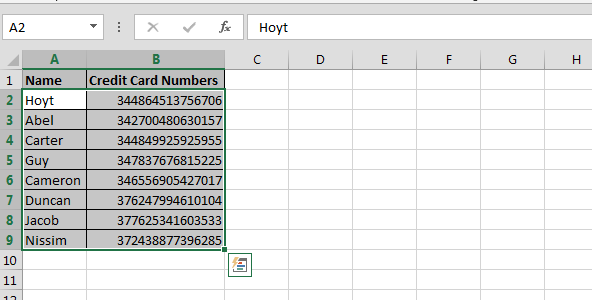• Copy the range by pressing the key Ctrl+C
• And then select the cell where you want to show the data in horizontal range
• Right click with the mouse
• Pop-up will appear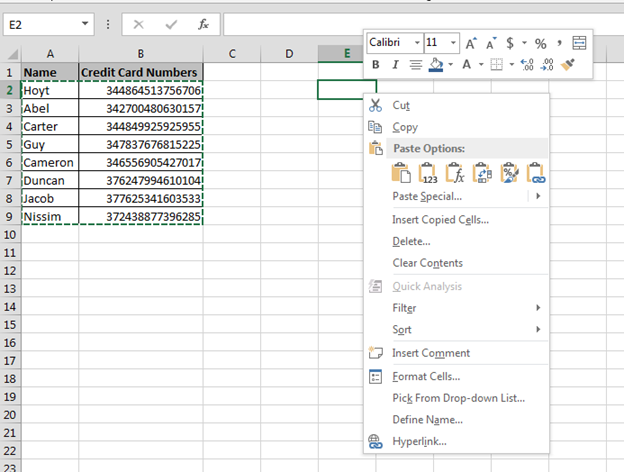• Select Paste-Special option
• Paste Special dialog box will appear
• Click on Transpose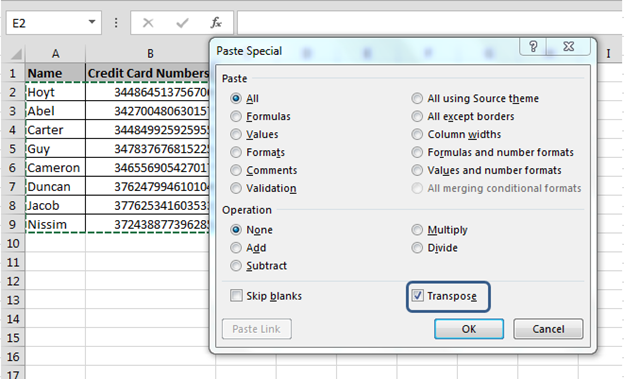• Data will get converted from Vertical range to horizontal range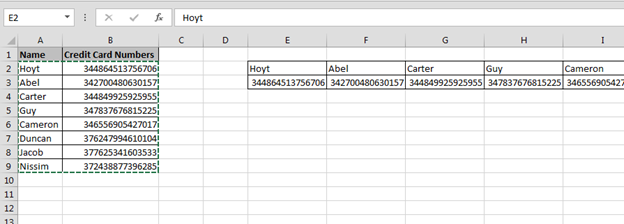This is called Transpose and these are the ways of using the Transpose function and Option in Microsoft Excel

Take away:-

• Paste for Transpose
• Excel function for Transpose

Below you can find more example to learn about Transpose:-

How to transposing Values from Columns into Rows, and Vice Versa

How to use Transpose function

How to Transpose in Microsoft Excel 2007

How to Copy Vertical and Paste Horizontal in Microsoft Excel1.